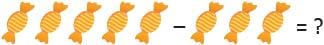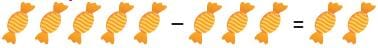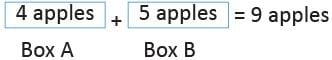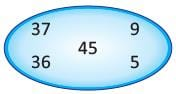Olympiad Test Level 1: Addition and Subtraction - 2

# Olympiad Test Level 1: Addition and Subtraction - 2

Test Description

## 15 Questions MCQ Test Math Olympiad for Class 2 | Olympiad Test Level 1: Addition and Subtraction - 2

Olympiad Test Level 1: Addition and Subtraction - 2 for Class 2 2022 is part of Math Olympiad for Class 2 preparation. The Olympiad Test Level 1: Addition and Subtraction - 2 questions and answers have been prepared according to the Class 2 exam syllabus.The Olympiad Test Level 1: Addition and Subtraction - 2 MCQs are made for Class 2 2022 Exam. Find important definitions, questions, notes, meanings, examples, exercises, MCQs and online tests for Olympiad Test Level 1: Addition and Subtraction - 2 below.
Solutions of Olympiad Test Level 1: Addition and Subtraction - 2 questions in English are available as part of our Math Olympiad for Class 2 for Class 2 & Olympiad Test Level 1: Addition and Subtraction - 2 solutions in Hindi for Math Olympiad for Class 2 course. Download more important topics, notes, lectures and mock test series for Class 2 Exam by signing up for free. Attempt Olympiad Test Level 1: Addition and Subtraction - 2 | 15 questions in 30 minutes | Mock test for Class 2 preparation | Free important questions MCQ to study Math Olympiad for Class 2 for Class 2 Exam | Download free PDF with solutions
 1 Crore+ students have signed up on EduRev. Have you?
Olympiad Test Level 1: Addition and Subtraction - 2 - Question 1

###Detailed Solution for Olympiad Test Level 1: Addition and Subtraction - 2 - Question 1Olympiad Test Level 1: Addition and Subtraction - 2 - Question 2

### There are 42 students in Class 2, Section A, 35 in Section B. How many total students are there in Section A and Section B ?

Detailed Solution for Olympiad Test Level 1: Addition and Subtraction - 2 - Question 2

Total students = 42 + 35 = 77

Olympiad Test Level 1: Addition and Subtraction - 2 - Question 3

### _______ – 21 = 24

Detailed Solution for Olympiad Test Level 1: Addition and Subtraction - 2 - Question 3

45 – 21 = 24

Olympiad Test Level 1: Addition and Subtraction - 2 - Question 4

Direction: Find the missing number.

82 – 46 = _________

Detailed Solution for Olympiad Test Level 1: Addition and Subtraction - 2 - Question 4

82 – 46 = 36

Olympiad Test Level 1: Addition and Subtraction - 2 - Question 5

Find the missing number.
22 + 9 = 11 + ________

Detailed Solution for Olympiad Test Level 1: Addition and Subtraction - 2 - Question 5

22 + 9 = 11 + 20

Olympiad Test Level 1: Addition and Subtraction - 2 - Question 6

How much should be subtracted from 60 to get 27.

Detailed Solution for Olympiad Test Level 1: Addition and Subtraction - 2 - Question 6

60 – 33 = 27

Olympiad Test Level 1: Addition and Subtraction - 2 - Question 7

Which of the following is equal to 8 + 12 + 16 ?

Detailed Solution for Olympiad Test Level 1: Addition and Subtraction - 2 - Question 7

8 + 12 + 16 = 36

Olympiad Test Level 1: Addition and Subtraction - 2 - Question 8

Ravi had 4 apples in Box A and 5 apples in Box B. How many total apples Ravi had ?

Detailed Solution for Olympiad Test Level 1: Addition and Subtraction - 2 - Question 8Olympiad Test Level 1: Addition and Subtraction - 2 - Question 9

What number is obtained on addition of 6 and 3 ?

Detailed Solution for Olympiad Test Level 1: Addition and Subtraction - 2 - Question 9

6 + 3 = 9

Olympiad Test Level 1: Addition and Subtraction - 2 - Question 10

Julia had 25 chocolates and Amelia had 65 chocolates. How many chocolates were there in all ?

Detailed Solution for Olympiad Test Level 1: Addition and Subtraction - 2 - Question 10

Total chocolates = 25 + 65 = 90 chocolates

Olympiad Test Level 1: Addition and Subtraction - 2 - Question 11

40 has the same value as :

Detailed Solution for Olympiad Test Level 1: Addition and Subtraction - 2 - Question 11

20 + 20 = 40

Olympiad Test Level 1: Addition and Subtraction - 2 - Question 12

Which mathematical statement gives same value as 31 - 23 ?

Detailed Solution for Olympiad Test Level 1: Addition and Subtraction - 2 - Question 12

23 – 15 = 8
21 – 13 = 8

Olympiad Test Level 1: Addition and Subtraction - 2 - Question 13

Which of the following is equal to 3 + 11 + 17 ?

Detailed Solution for Olympiad Test Level 1: Addition and Subtraction - 2 - Question 13

3 + 11 + 17 = 31
41 – 10 = 31

Olympiad Test Level 1: Addition and Subtraction - 2 - Question 14

Find out the addition of the numbers given inside the circle.Detailed Solution for Olympiad Test Level 1: Addition and Subtraction - 2 - Question 14

37 + 36 + 45 + 9 + 5
= 132

Olympiad Test Level 1: Addition and Subtraction - 2 - Question 15

Which of the following option is more than 7 + 5 but less than 8 + 6 ?

Detailed Solution for Olympiad Test Level 1: Addition and Subtraction - 2 - Question 15

10 + 3 = 13
7 + 6 = 13
8 + 5 = 13

## Math Olympiad for Class 2

72 tests
 Use Code STAYHOME200 and get INR 200 additional OFF Use Coupon Code
Information about Olympiad Test Level 1: Addition and Subtraction - 2 Page
In this test you can find the Exam questions for Olympiad Test Level 1: Addition and Subtraction - 2 solved & explained in the simplest way possible. Besides giving Questions and answers for Olympiad Test Level 1: Addition and Subtraction - 2, EduRev gives you an ample number of Online tests for practice

72 tests